• Convertible notes

# Convertible notes - Are you accounting for these correctly (Part 3)?

In the current economic climate, we continue to see different types of convertible note arrangements, typically entered into by companies needing to offer attractive returns in order to obtain funds from lenders and investors.

Last month, our Accounting Alert article worked through an example of a convertible note classified as a compound financial instrument (i.e. part debt and part equity). This month, we look at an example of a convertible note with an embedded derivative liability.

Why do some conversion features fail the “fixed for fixed” test to be classified as equity?

In practice, many conversion features in convertible notes fail equity classification, which means that the conversion feature is a financial liability.

 The reason for this is that the conversion feature contains contractual terms that result in the holder of the conversion feature having rights that are different to those of existing shareholders.

This could occur because the contractual terms are such that:

• The number of shares to be issued varies
• The amount of cash (or carrying amount of the liability) converts into a variable number of shares, or
• Both the number of shares, and the amount of cash (the carrying amount of the liability), vary.

The commercial effect of this is that the holder of the conversion feature obtains a different return in comparison with an investor that holds equity shares.

Conversion features that fail equity classification are derivatives

Conversion features that fail equity classification are derivatives because they are either:

• Written options (i.e. options that provide the holder with a choice over whether the convertible note is exchanged for shares or cash), or
• Forward contracts under which an entity will issue shares in order to extinguish an obligation, with no cash settlement alternative.

This links to the definition of a derivative in IAS 39, with all three of the characteristics of a derivative being met, i.e.:

• The value of the conversion feature changes in response to the share price of the issuer
• The investment required to purchase the option is less than would be required to purchase the equivalent number of shares1, and
• The conversion feature can or will be exercised at a future date.
1. In this context, the “investment required to purchase the options” is calculated as the present value of the reduction in interest rate paid on the convertible note compared to a loan note with no conversion feature. This amount is less than the “amount required to purchase the equivalent number of shares”. Therefore, we compare the cost of acquiring the conversion feature with the cost of acquiring shares themselves.

How do we account for conversion features that are derivatives?

There are two ways that derivative conversion features can be accounted for:

1. At fair value through profit or loss, separately from the host debt contract, or
2. Using the fair value option in IAS 39 Financial Instruments: Recognition and Measurement (or IFRS 9 Financial Instruments (“IFRS 9”) post 1 January 2018).

Option 1 – Account for the conversion feature at fair value through profit or loss separately from host debt contract

Conversion features that fail equity classification and are accounted for as derivative liabilities are typically accounted for separately from the host instruments.  This is because the fair value of the conversion feature is affected by changes in the fair value of the issuer’s shares, and the fair value of the host loan is not.  This means that the conversion feature (an embedded derivative) is not what IAS 39/IFRS 9 refer to as being “closely related” to the host contract.

The effect of this option is that the instrument is separated into two components and accounted for as follows: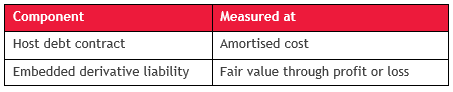Option 2 – Fair value for whole note

The terms of some convertible notes which include an embedded derivative liability can mean that accounting for each of the separate components can become complex.

IAS 39 and IFRS 9 therefore both contain a fair value option. Rather than just determining the fair value of the derivative, the fair value is determined for the convertible note in its entirety. Fair value movements from one period to another are then recognised in profit or loss.

Although this may appear to be an attractive option, it can give rise to additional volatility in amounts reported in profit or loss.  This is because both the embedded derivative(s) and also the host loan will be measured at fair value, with the fair value of the host contract being affected by factors such as changes in interest rates, and changes in the issuer’s own credit rating, which would not be accounted for if it had been measured at amortised cost.

How to separate the convertible note (Option 1)?

Assuming we choose Option 1 above, at initial recognition, the proceeds received on issue of the convertible note need to be split between the host debt contract and the embedded derivative liability. In subsequent periods, the host debt contract continues to be measured at amortised cost, and the embedded derivative liability is measured at fair value through profit or loss.

 The process of separating an embedded derivative liability from a host debt contract is different to that applied when separating an equity conversion feature, which we illustrated in our May Accounting Alert article. In that case, for a compound instrument, we calculate the debt portion first, and the equity portion is the residual amount.

The accounting for a convertible note with an embedded derivative liability is set out in IAS 39, paragraph AG28 (IFRS 9, paragraph B4.3.3).

 An embedded non-option derivative (such as an embedded forward or swap) is separated from its host contract on the basis of its stated or implied substantive terms, so as to result in it having a fair value of zero at initial recognition. An embedded option-based derivative (such as an embedded put, call, cap, floor or swaption) is separated from its host contract on the basis of the stated terms of the option feature. The initial carrying amount of the host instrument is the residual amount after separating the embedded derivative. IAS 39, paragraph AG28/IFRS 9, paragraph B.4.3.3

The embedded derivative liability is calculated first and the residual value is assigned to the debt host liability component (IAS 39, paragraph AG28).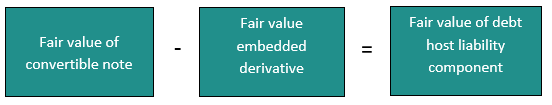Example

Entity A issues a note with a face value of \$1,000, maturing three years from its date of issue.

The note pays a 10% annual coupon.

On maturity at the end of three years, the holder has an option either to receive a cash repayment of \$1,000 or to convert the note into Entity A’s shares.

The number of shares to be issued on conversion is calculated using the average of the lowest five days’ value weighted average price (“VWAP”) in the previous 30 days prior to maturity.  The conversion feature is determined to have a fair value of \$20 at issue date.

Note: This example does not deal with the allocation of transaction costs to the host debt and derivative liability components.

Using the flowchart below for the entire instrument, we conclude that the convertible note is a financial liability with an embedded derivative liability.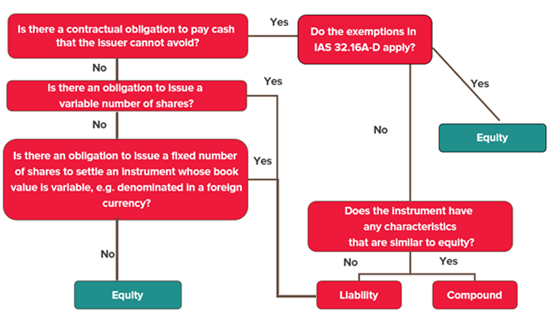Step one

Starting with the box on the top left hand side of the flowchart above, we consider whether there is a contractual obligation to pay cash that Entity A (the issuer) cannot avoid.  The answer is yes, because it pays an annual cash coupon, and could be required to repay the capital amount at the end of three years if the holder chooses not to exercise the conversion option.

Step two

Step 2 is to consider whether paragraphs 16A to 16D of IAS 32 Financial Instruments: Presentation (“IAS 32”) apply.  When an issuer has an obligation to repurchase financial instruments in certain circumstances, paragraphs 16A to 16D of IAS 32 include exceptions to the usual principles for classifying instruments as financial liabilities. In some cases, such instruments could be classified as equity, despite an entity having an unavoidable obligation to pay out cash. However, this exception does not typically apply to convertible instruments and is not applicable in this example.

Step three

Continuing down the right hand side of the above flow chart, we then consider whether the instrument has any characteristics that are similar to equity.  The answer is yes because the instrument contains an option to be converted into equity instruments, but the question of whether the conversion feature meets the criteria to be classified as equity is dealt with separately in Step 4 below.

The host debt component will be classified as a financial liability in its entirety because of Entity A’s unavoidable obligation to pay cash, and on a stand-alone basis there is no feature in the host debt instrument that is similar to equity.

Step four

The conversion feature is then assessed, again on a stand-alone basis.  Starting with the box at the top left hand side of the diagram:

• There is no unavoidable contractual obligation to pay cash by the issuer. The equity conversion feature can only be settled through the issue of equity shares, otherwise it will simply expire unexercised.
• There is an obligation to issue a variable number of shares – the number of shares to be issued is based on the lowest 5 day VWAP in the last 30 days prior to maturity.

Therefore, the conversion feature is also classified as a financial liability. The conversion feature also meets the definition of a derivative financial liability because:

• The value of the conversion feature changes in response to the share price of the issuer (the value of the conversion feature is dependent on the 5 day VWAP and also the share price on date of conversion)
• The investment required to purchase the option is less than would be required to purchase the equivalent number of shares, and
• The conversion feature can or will be exercised at a future date (in three years’ time).

This means that the note contains the following components:

• Contractual cash flows of 10% annual coupons and a cash repayment of \$1,000 (liability)
• The conversion feature to convert the liability into equity of Entity A at the lowest five day VWAP in the previous 30 days prior to maturity (an embedded derivative liability).

For convertible notes with embedded derivative liabilities, the embedded derivative liability is determined first and the residual value is assigned to the debt host liability.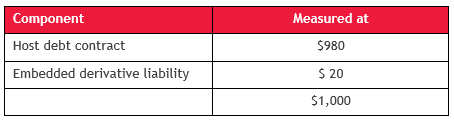Therefore, the debt host liability is initially recognised at \$980 being the residual value from deducting the fair value of the derivative liability from the transaction price (i.e. \$1,000 less \$20).

Subsequent measurement

At subsequent reporting dates, the host debt contract continues to be measured at amortised cost with interest expense recognised using the effective interest method. However, the fair value of the conversion feature (derivative liability) must be determined at each reporting date and the fair value changes recognised in profit or loss.

The following table sets out the effect on profit or loss assuming the following fair values at each year end: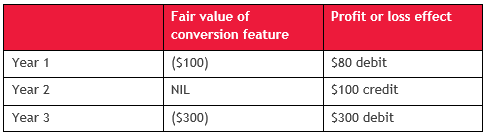Therefore we would expect more profit or loss volatility for conversion features classified as derivative liabilities.

If the conversion feature is classified as a derivative liability, this will often lead to a significantly higher and more volatile expense pattern in trading profit or loss.  This is because a derivative liability is remeasured to fair value at each reporting date, whereas if the conversion feature is classified as equity, there is no re-measurement of the conversion feature.

IFRS 13 Fair Value Measurement (“IFRS 13”) requires an entity to take into account the effects of its own non-performance risk or credit risk (“own credit”) when measuring the fair value of a liability (IFRS 13, paragraphs 42 and 43). This requirement also applies to embedded derivative liabilities that arise as a result of issuing convertible notes. For entities that have used the fair value option (Option 2 above), IFRS 9 requires entities to recognise the changes in own credit in other comprehensive income, rather than profit or loss.

Fair value hierarchy and disclosures

IFRS 13 contains extensive disclosure requirements regarding fair value. The standard establishes a three level hierarchy for categorising inputs used to measure fair value:

• Level 1: Quoted prices (unadjusted) in active markets
• Level 2: Inputs other than quoted prices that are either directly or indirectly observable
• Level 3: Unobservable inputs.

Measurements with level 1 inputs require less disclosure, while those with level 3 inputs require the most disclosure (e.g. for level 3 fair value measurements, IFRS 13 requires entities to quantify all significant unobservable inputs (IFRS 13, paragraph 93(d)) and to quantify sensitivity analyses (IFRS 13, paragraph 93(h)(ii)). For a convertible note that contains an embedded derivative liability feature, consideration needs to be given to whether the embedded derivative liability falls within level 2 or level 3 of the fair value hierarchy.

Next month

Next month, we will look at some scenarios where conversion features fail equity classification, and also where they still meet equity classification.